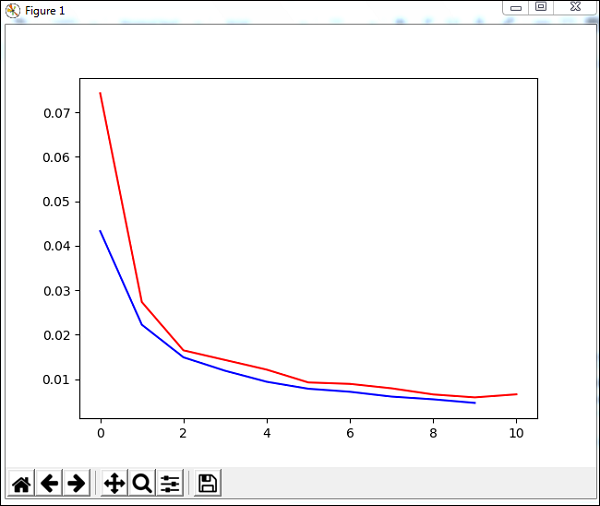# PyBrain - Testing Network

In this chapter, we are going to see some example where we are going to train the data and test the errors on the trained data.

We are going to make use of trainers −

### BackpropTrainer

BackpropTrainer is trainer that trains the parameters of a module according to a supervised or ClassificationDataSet dataset (potentially sequential) by backpropagating the errors (through time).

### TrainUntilConvergence

It is used to train the module on the dataset until it converges.

When we create a neural network, it will get trained based on the training data given to it.Now whether the network is trained properly or not will depend on prediction of test data tested on that network.

Let us see a working example step by step which where will build a neural network and predict the training errors, test errors and validation errors.

## Testing our Network

Following are the steps we will follow for testing our Network −

• Importing required PyBrain and other packages
• Create ClassificationDataSet
• Splitting the datasets 25% as testdata and 75% as trained data
• Converting Testdata and Trained data back as ClassificationDataSet
• Creating a Neural Network
• Training the Network
• Visualizing the error and validation data
• Percentage for test data Error

Step 1

Importing required PyBrain and other packages.

The packages that we need are imported as shown below −

from sklearn import datasets
import matplotlib.pyplot as plt
from pybrain.datasets import ClassificationDataSet
from pybrain.utilities import percentError
from pybrain.tools.shortcuts import buildNetwork
from pybrain.supervised.trainers import BackpropTrainer
from pybrain.structure.modules import SoftmaxLayer
from numpy import ravel


Step 2

The next step is to create ClassificationDataSet.

For Datasets, we are going to use datasets from sklearn datasets as shown below −

digits = datasets.load_digits()
X, y = digits.data, digits.target

ds = ClassificationDataSet(64, 1, nb_classes=10)
# we are having inputs are 64 dim array and since the digits are from 0-9 the
classes considered is 10.

for i in range(len(X)):


Step 3

Splitting the datasets 25% as testdata and 75% as trained data −

test_data_temp, training_data_temp = ds.splitWithProportion(0.25)


So here, we have used a method on dataset called splitWithProportion() with value 0.25, it will split the dataset into 25% as test data and 75% as training data.

Step 4

Converting Testdata and Trained data back as ClassificationDataSet.

test_data = ClassificationDataSet(64, 1, nb_classes=10)
for n in range(0, test_data_temp.getLength()):
training_data = ClassificationDataSet(64, 1, nb_classes=10)

for n in range(0, training_data_temp.getLength()):

training_data_temp.getSample(n), training_data_temp.getSample(n)
)
test_data._convertToOneOfMany()
training_data._convertToOneOfMany()


Using splitWithProportion() method on dataset converts the dataset to superviseddataset, so we will convert the dataset back to classificationdataset as shown in above step.

Step 5

Next step is creating a Neural Network.

net = buildNetwork(training_data.indim, 64, training_data.outdim, outclass=SoftmaxLayer)


We are creating a network wherein the input and output are used from the training data.

Step 6

### Training the Network

Now the important part is training the network on the dataset as shown below −

trainer = BackpropTrainer(net, dataset=training_data,
momentum=0.1,learningrate=0.01,verbose=True,weightdecay=0.01)


We are using BackpropTrainer() method and using dataset on the network created.

Step 7

The next step is visualizing the error and validation of the data.

trnerr,valerr = trainer.trainUntilConvergence(dataset=training_data,maxEpochs=10)
plt.plot(trnerr,'b',valerr,'r')
plt.show()


We will use a method called trainUntilConvergence on training data that will converge for epochs of 10. It will return training error and validation error which we have plotted as shown below. The blue line shows the training errors and red line shows the validation error.Total error received during execution of the above code is shown below −

Total error: 0.0432857814358
Total error: 0.0222276374185
Total error: 0.0149012052174
Total error: 0.011876985318
Total error: 0.00939854792853
Total error: 0.00782202445183
Total error: 0.00714707652044
Total error: 0.00606068893793
Total error: 0.00544257958975
Total error: 0.00463929281336
Total error: 0.00441275665294
('train-errors:', '[0.043286 , 0.022228 , 0.014901 , 0.011877 , 0.009399 , 0.007
822 , 0.007147 , 0.006061 , 0.005443 , 0.004639 , 0.004413 ]')
('valid-errors:', '[0.074296 , 0.027332 , 0.016461 , 0.014298 , 0.012129 , 0.009
248 , 0.008922 , 0.007917 , 0.006547 , 0.005883 , 0.006572 , 0.005811 ]')


The error starts at 0.04 and later goes down for each epoch, which means the network is getting trained and gets better for each epoch.

Step 8

### Percentage for test data error

We can check the percent error using percentError method as shown below −

print('Percent Error on
testData:',percentError(trainer.testOnClassData(dataset=test_data),
test_data['class']))


Percent Error on testData − 3.34075723830735

We are getting the error percent, i.e., 3.34%, which means the neural network is 97% accurate.

Below is the full code −

from sklearn import datasets
import matplotlib.pyplot as plt
from pybrain.datasets import ClassificationDataSet
from pybrain.utilities import percentError
from pybrain.tools.shortcuts import buildNetwork
from pybrain.supervised.trainers import BackpropTrainer
from pybrain.structure.modules import SoftmaxLayer
from numpy import ravel
X, y = digits.data, digits.target

ds = ClassificationDataSet(64, 1, nb_classes=10)

for i in range(len(X)):

test_data_temp, training_data_temp = ds.splitWithProportion(0.25)
test_data = ClassificationDataSet(64, 1, nb_classes=10)
for n in range(0, test_data_temp.getLength()):
training_data = ClassificationDataSet(64, 1, nb_classes=10)

for n in range(0, training_data_temp.getLength()):
training_data_temp.getSample(n), training_data_temp.getSample(n)
)
test_data._convertToOneOfMany()
training_data._convertToOneOfMany()

net = buildNetwork(training_data.indim, 64, training_data.outdim, outclass=SoftmaxLayer)
trainer = BackpropTrainer(
net, dataset=training_data, momentum=0.1,
learningrate=0.01,verbose=True,weightdecay=0.01
)
trnerr,valerr = trainer.trainUntilConvergence(dataset=training_data,maxEpochs=10)
plt.plot(trnerr,'b',valerr,'r')
plt.show()

trainer.trainEpochs(10)
print('Percent Error on testData:',percentError(
trainer.testOnClassData(dataset=test_data), test_data['class']
))